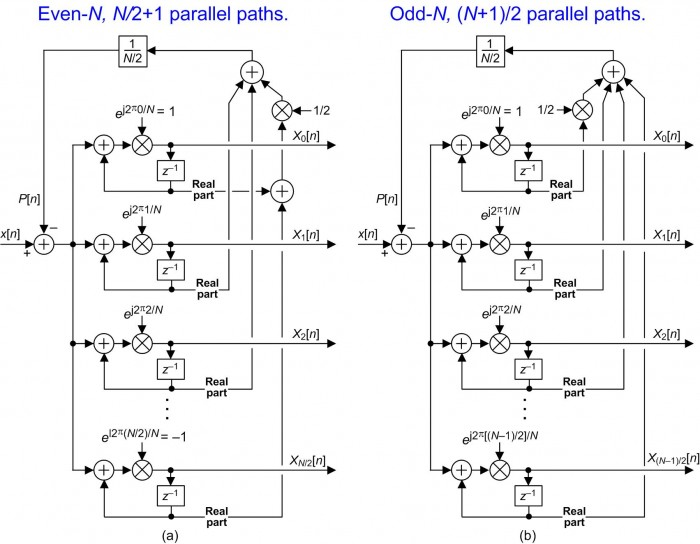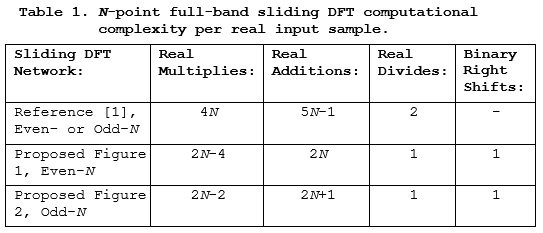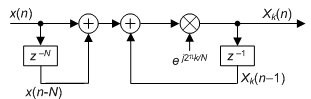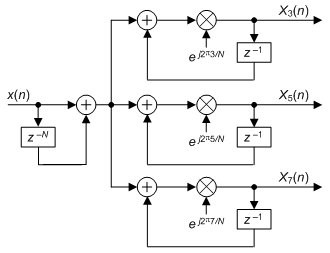# An Efficient Full-Band Sliding DFT Spectrum Analyzer

In this blog I present two computationally efficient full-band discrete Fourier transform (DFT) networks that compute the 0th bin and all the positive-frequency bin outputs for an N-point DFT in real-time on a sample-by-sample basis.

An Even-N Spectrum Analyzer

The full-band sliding DFT (SDFT) spectrum analyzer network, where the DFT size N is an even integer, is shown in Figure 1(a). The x[n] input sequence is restricted to be real-only valued samples. Notice that the only real parts of the delay elements’ complex-valued outputs are used to compute the feedback P[n] samples.The Figure 1(a) network’s complex coefficients are defined by $e^{j2{\pi}k/N}$ where integer k defines the DFT bin index and is in the range 0 ≤ kN/2. This even-N network has N/2+1 parallel paths that compute the complex-valued X0, X1, X2,..., XN/2 DFT bin output samples. The multiply by 1/2 can be implemented with a simple binary right shift by one bit.

The Figure 1(a) even-N network was inspired by the Figure 4 network in Reference . However, for real-valued input samples I’ve simplified the Reference  network (which has N parallel paths) and reduced its computational complexity to develop the above proposed Figure 1(a) network.

An Odd-N Spectrum Analyzer

To compute real-time positive-frequency odd-N-point DFT spectral samples we use the network shown in Figure 1(b). This odd-N network has (N+1)/2 parallel paths that compute the X0, X1, X2,..., X(N-1)/2 DFT bin output samples. Again the x[n] input sequence is restricted to be real-only valued samples. The Figure 1(b) network’s complex coefficients are defined by $e^{j2{\pi}k/N}$ where integer k is 0 ≤ k ≤ (N-1)/2.

Two surprising properties of both Figure 1 networks are: 1) although they use recursive complex multiplications the networks are guaranteed stable, and 2) the networks compute sliding DFT samples without a comb delay-line section required by traditional sliding DFT networks! (The Reference  network also has those two noteworthy properties.)

Implementation

Both Figure 1 networks are implemented as follows:

1) Set the outputs of the delay elements and the P feedback sample to zero.

2) Accept the first x input sample and compute the Xk output samples for each parallel path.

3) Shift the data through the delay elements and compute the P feedback sample.

4) Accept the second input sample and compute the Xk output samples for each parallel path.

5) Shift the data through the delay elements and compute the new P feedback sample.

6) And so on.

Computational Complexity Comparison

In case you’re interested in the computational workload of the Reference  network and our two proposed Figure 1 networks, have a look at Table 1.Conclusion

I presented computationally efficient full-band sliding DFT networks that compute the 0th bin and all the positive-frequency DFT bin outputs for both even- and odd-N-point DFTs in real-time on a sample-by-sample basis. (No taxpayer money was used and no animals were injured in the creation of this blog.)References

 Z. Kollar, F. Plesnik, and S. Trumpf, “Observer-Based Recursive Sliding Discrete Fourier Transform,” IEEE Signal Proc. Mag., Vol. 35, No. 6, pp. 100-106, Nov. 2018.

[ - ]
Comment by April 9, 2021I notice this is posted on April 1.  Am I foolish to read it?

John P

[ - ]
Comment by April 9, 2021Hi JProvidenza.

Ah ha. Such an interesting question. The answer is no, you would not be foolish to read it. (Perhaps I should have paid more attention to the date and posted this blog on April 2nd.)

[ - ]
Comment by April 11, 2021Rick -

I couldn't resist the comment - it was too sweet a date!

Thanks for all your great posts.  I have a couple of your books that I've used for study - my background is not DSP, but it's great to stretch my brain on topics I was not introduced to in college decades ago.

John P

[ - ]
Comment by April 13, 2021Rick -

Could you add a little info on how to interpret the output?

Thanks!

John P

[ - ]
Comment by April 14, 2021Hi JProvidenza.

Well, ...the traditional sliding DFT, shown in the following diagram, is used to compute the real-time (one output sample for each new input sample) kth bin output of a N-point DFT. (The X_k[n] output is the kth bin output of a N-point DFT for the current input sample and the previous N-1 input samples.) The typical application of the traditional sliding DFT is to detect the presence, or absence, of spectral energy centered at the kth bin of an N-point DFT in real time.If you wanted to simultaneously compute the k = 3, k = 5, and k = 7 bins’ outputs you would use the following network.For real-valued x[n] input sequences, my blog’s Figure 1 networks are used to compute the 0th bin and all the positive-frequency bin outputs of an N-point DFT in real-time (on a sample-by-sample basis). Notice in my blog’s Figure 1 that, unlike the traditional sliding DFT, no N-length delay line stage is needed.

I hope what I've written here makes sense.

[ - ]
Comment by November 5, 2021Hi Rick,

I am not sure if my question is related to this exact article.

Could the sliding DFT be used to extract different bins of an OFDM signal which is not really like a sine wave. Also is it applicable on parallel samples?

Regards,

Tushar

[ - ]
Comment by November 5, 2021Hello tushar_tyagi90

This blog presents an efficient way to compute the N complex output bin samples of an N-point DFT in real time. By “real time” I mean that that for each data input sample all N DFT bin complex spectral sample values are computed. The output samples of this scheme are not valid until the Nth input sample has been applied to the network. [The traditional DFT (or radix-2 FFT) provides outputs only for every N input samples--it’s not “real-time”.] The networks in this blog's Figure 1 are only valid for real-valued input samples.

A sliding DFT network, on the other hand, computes a single complex output bin spectral sample of an N-point DFT in real time (one complex output spectral sample for each input sample).

I have no experience in OFDM processing, but if your OFDM processing requires you to compute all N output spectral samples of an N-point DFT in real time then one of the networks in this blog’s Figure 1 should achieve your goal.

If your OFDM processing requires you to compute, say 8, real time N-point DFT spectral samples (where N is greater than 8) then you merely need to implement 8 sliding DFT networks. That is, one comb section driving 8 separate resonator sections.

I must tell you, “traditional” sliding DFT networks can experience long-term stability problems. Several guaranteed-stable sliding DFT networks have been proposed in the literature but they’re more computationally intensive than the traditional sliding DFT. Last year I developed a guaranteed-stable sliding DFT network that is significantly more computationally efficient than the previously proposed guaranteed-stable sliding DFT networks.

My “new and improved” guaranteed-stable sliding DFT is described at:

"Improvements to the Sliding DFT Algorithm", DSP Tips & Tricks column, IEEE Signal Processing Magazine, Vol. 38, No. 4, July 2021.

I intend to write a blog at this dsprelated.com web site describing my new and improved sliding DFT network as soon as I have the time.

tushar_tyagi90, if you have any further questions for me please let me know.

To post reply to a comment, click on the 'reply' button attached to each comment. To post a new comment (not a reply to a comment) check out the 'Write a Comment' tab at the top of the comments.